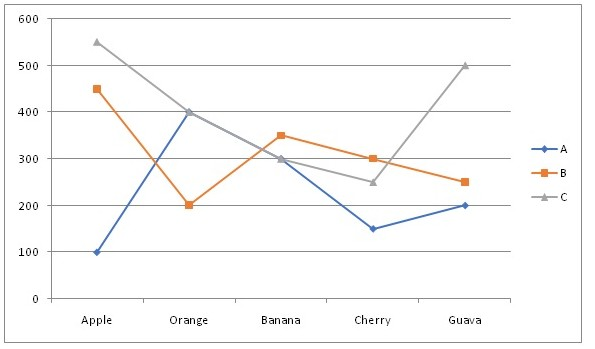# SBI PO Prelims Quantitative Aptitude (Day-01)

Dear Aspirants, Our IBPS Guide team is providing new series of Quantitative Aptitude Questions for SBI PO 2020 so the aspirants can practice it on a daily basis. These questions are framed by our skilled experts after understanding your needs thoroughly. Aspirants can practice these new series questions daily to familiarize with the exact exam pattern and make your preparation effective.

Start Quiz

Directions (1-5): Study the following information carefully and answer the questions given below.

The given line graph shows the number of fruits sold in three different shops A, Band C.1) The number of Cherry sold in C is what percent of the total number of Banana sold in A and B together?

A.29.5%

B.33.8%

C.38.4%

D.41.2%

E.None of these

2) What is the ratio of the average number of fruits sold in A to B?

A.23:31

B.23:34

C.11:15

D.19:30

E.None of these

3) The number of Banana, Orange and Guava together sold in A is what percent of the number of Apple, Cherry and Orange together sold in C?

A.50%

B.65%

C.70%

D.75%

E.60%

4) If the number of Mango sold by A is 40% more than the number of Guava sold in C and the number of mango sold in B is 25% more than the number of Orange sold in A and the number of Mango sold in C is 80% of the total number of Cherry sold in B, then what is the total number of Mango sold in A, B and C together?

A.1380

B.1440

C.1520

D.1600

E.None of these

5) If each fruit of Apple, Orange, Banana, Cherry and Guava sold in A at Rs.30, Rs.25, Rs.15, Rs.20 and Rs.10 respectively, then find the revenue collected from shop A.

A.Rs.22500

B.Rs.19600

C.Rs.18900

D.Rs.24600

E.None of these

Directions (6-10):Following question contains two equations as I and II. You have to solve both equations and determine the relationship between them and give answer as,

6)

I) x2+ 9x + 20 = 0

II) y2+ 13y + 42 = 0

A. x > y

B. x ≥ y

C. x = y or relationship can’t be determined.

D. x < y

E. x ≤ y

7)

I) x2– 18x + 72 = 0

II) y2– 21y + 108 = 0

A. x > y

B. x ≥ y

C. x = y or relationship can’t be determined.

D. x < y

E. x ≤ y

8)

I) 2x2– 2x – 84 = 0

II) y2– 2y – 48 = 0

A. x > y

B. x ≥ y

C. x = y or relationship can’t be determined.

D. x < y

E. x ≤ y

9)

I) 2x + y = 34

II) x + 3y = 47

A. x > y

B. x ≥ y

C. x = y or relationship can’t be determined.

D. x < y

E. x ≤ y

10)

I) x2+ 32x – 144 = 0

II) y2– 31y + 108 = 0

A. x > y

B. x ≥ y

C. x = y or relationship can’t be determined.

D. x < y

E. x ≤ y

Directions (1-5):

Required percentage = [250/(300 + 350)] * 100 = 38.4%

Required ratio = (100 + 400 + 300 + 150 + 200)/5:(450 + 200 + 350 + 300 + 250)/5

= 1150/5:1550/5

=23:31

Required percentage = (300 + 400 + 200)/(550 + 250 + 400) * 100 = 75%

Number of Mango sold in A = 140/100 * 500 = 700

Number of Mango sold in B = 125/100 * 400 = 500

Number of Mango sold in C = 80/100 * 300 = 240

Required sum = 700 + 500 + 240 = 1440

Total revenue collected from A = 30 * 100 + 400 * 25 + 300 * 15 + 150 * 20 + 200 * 10= Rs.22500

Directions (6-10):

x2 + 9x + 20 = 0

x2 + 4x + 5x + 20 = 0

x(x + 4) + 5(x + 4) = 0

(x + 5)(x + 4) = 0

x = -5, -4

y2 + 13y + 42 = 0

y2 + 6y + 7y + 42 = 0

y(y + 6) + 7(y + 6) = 0

(y + 7)(y + 6) = 0

y = -7, -6

x > y

x2 – 18x + 72 = 0

x2 – 12x – 6x + 72 = 0

x(x – 12) – 6(x – 12) = 0

x = 6, 12

y2 – 21y + 108 = 0

y2 – 12y – 9y + 108 = 0

y(y – 12) – 9(y – 12) = 0

(y – 9)(y – 12) = 0

y = 9, 12

Relationship between x and y cannot be established.

2x2 – 2x – 84 = 0

2x2 – 14x + 12x – 84 = 0

2x(x – 7) + 12(x – 7) = 0

(2x + 12)(x – 7) = 0

x = -6, 7

y2 – 2y – 48 = 0

y2 – 8y + 6y – 48 = 0

y(y – 8) + 6(y – 8) = 0

(y + 6)(y – 8) = 0

y = -6, 8

Relationship between x and y cannot be established.

2x + y = 34 ——–(1)

x + 3y = 47 —–(2)

(1) – (2) * 2

-5y = -60

y = 12

x = 47 – 36 = 11

x < y

x2 + 32x – 144 = 0

x2 + 36x – 4x – 144 = 0

x(x + 36) – 4(x + 36) = 0

(x – 4)(x + 36) = 0

x = 4, -36

y2 – 31y + 108 = 0

y2 – 27y – 4y + 108 = 0

y(y – 27) – 4(y – 27) = 0

(y – 4)(y – 27) = 0

y = 4, 27

x ≤ y